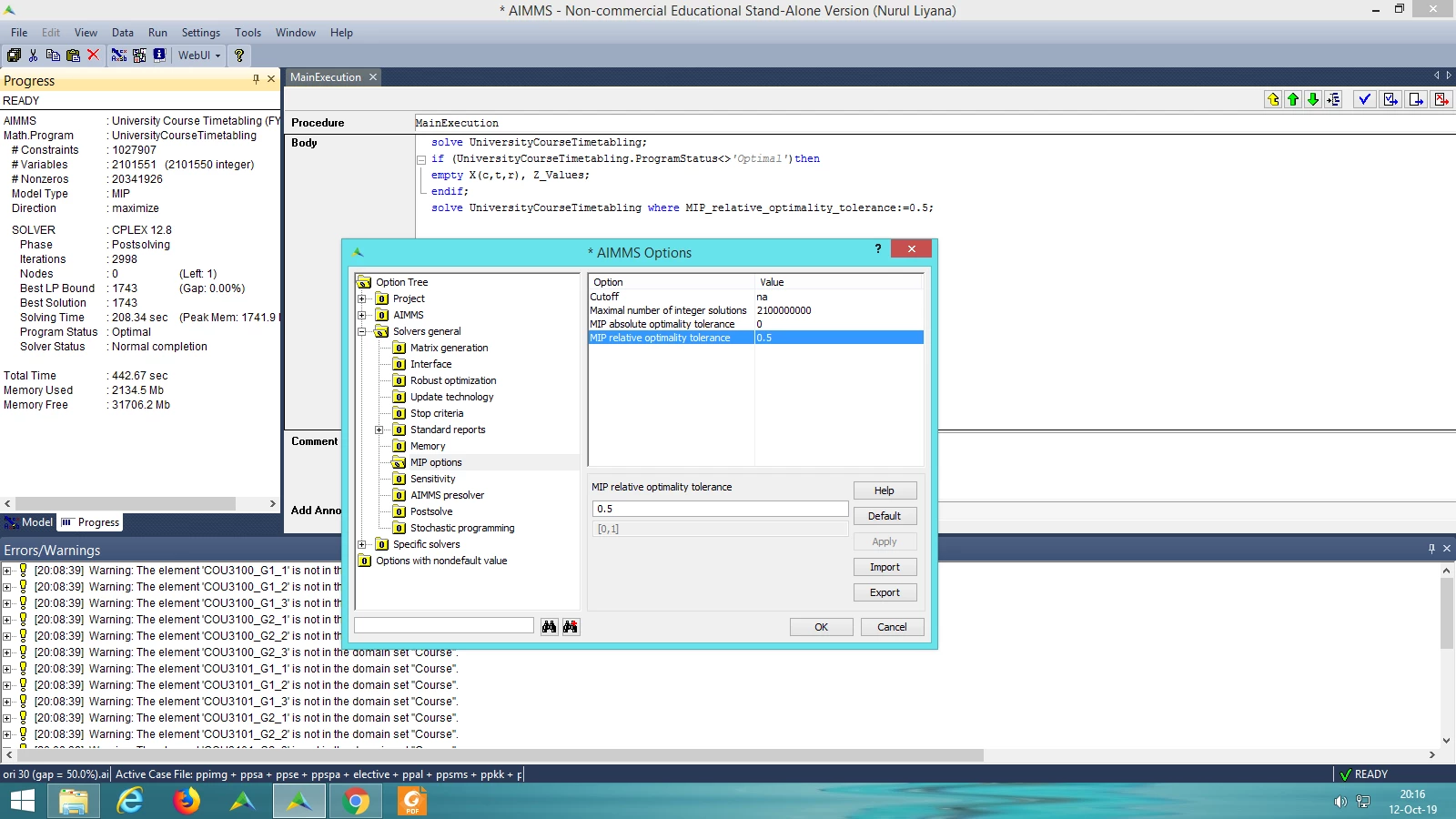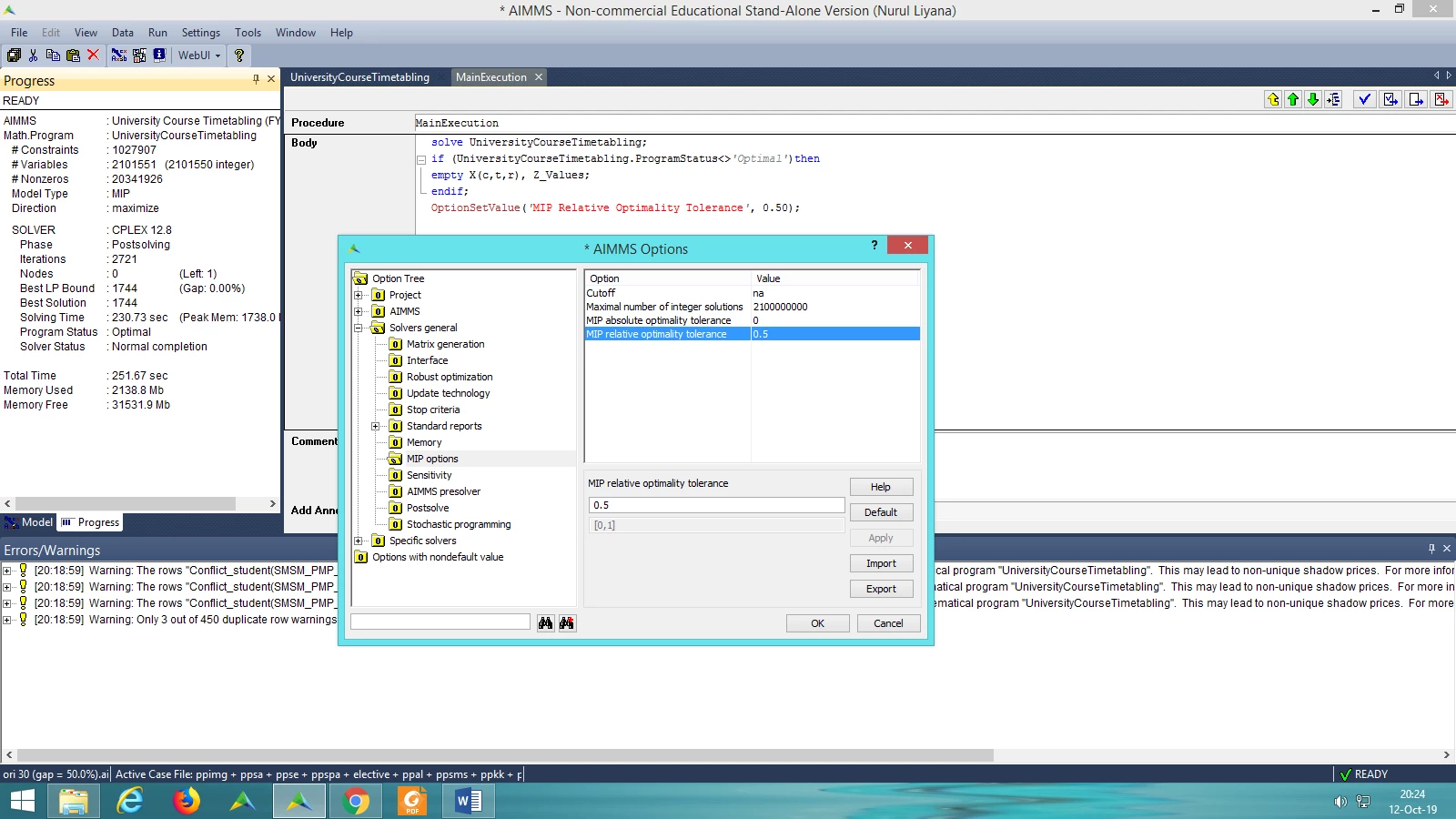Solved

# MIP Relative Optimality Tolerance

• Whiz
• 16 replies
Hi,

In the Options Tree, I have been changing the MIP Relative Optimality Tolerance to 0.5%, 1.0%, 5.0% and 10.0% to analyse the performance of the solution, gap and solving time.

I wish to change the gap to 0.5%, 1.0%, 5.0% and 10.0% to analyse whether the solving time can be reduce by changing the gap. However, the CPLEX always stop at either 0.04%, 0.12% or 0.00% of gap. Is there any way to stop the CPLEX at 10% of gap?
icon

Best answer by Marcel Hunting 16 October 2019, 17:14

View original

### 16 replies

Userlevel 5+2
You can try to set the tolerance within your optimization procedure through a code as well:

code:
`OptionSetValue('MIP Relative Optimality Tolerance', 0.10);`

Your model might be finding the first integer feasible solution with 0.00%/0.04% GAP.

I would also check if the model type in your Mathematical Program Identifier is set to MIP.
Hi.

I have try the code that you mentioned. I also have tried these methods:

1. Mathematical Program Identifier is set to MIP
2. changing the MIP Relative Optimality Tolerance in the Options Tree
3. and lastly:
code:
`solve MathProg where MIP_Relative_Optimality_Tolerance := 0.1;`

However, the CPLEX stop at 0.00%. Is there any way to stop the CPLEX at 10% of gap?
Hi @mateusarakawa ,

I have try the code that you mentioned. I also have tried these methods:

1. Mathematical Program Identifier is set to MIP
2. changing the MIP Relative Optimality Tolerance in the Options Tree
3. and lastly:
code:
`solve MathProg where MIP_Relative_Optimality_Tolerance := 0.1;`

However, the CPLEX stop at 0.00%. Is there any way to stop the CPLEX at 10% of gap?
Userlevel 5+2
@Zahidah Mohd Zaulir

Like @mateusarakawa mentioned, it might be that the first integer solution CPLEX is finding has gap 0. It is usually unlikely for this to happen but it is not impossible.

Can you share a screenshot of the progress window after this solve ? CTRL+P to open the progress window. Looking at the number of iterations, time it to reach optimality will help in finding out what's happening here.

Also, you do not need both "changing the MIP Relative Optimality Tolerance in the Options Tree" and "using the where keyword in the solve statement". Using solve mathprogram where .... would be better for your use case as changing the options tree will apply that option value to all solve statements in your project.
Hi @mohansx ,

Before you mention this, i did set both "changing the MIP Relative Optimality Tolerance in the Options Tree" and "using the where keyword in the solve statement". Attached together is the screenshot of the progress window and the two setting that i change.

For your information, right now I'm trying to re-run the model with only one setting, which is using the where keyword in the solve statement as you suggested. I will share the latest progress window later after the AIMMS stop running.

By the way, can you explain this to me:
1. In the progress window, what exactly is means by (Gap = 0.04%)? is it 0.04% of optimality gap, or 4% of optimality gap, or something else?
2. and if it is 4% of optimality gap, does it means that 96% of it is the best solution, meanwhile 4% of it is the less best solution?
Thanks for your help, I really appreciate it.Userlevel 5+2

Hi@Zahidah Mohd Zaulir.

In the progress window, what exactly is means by (Gap = 0.04%)? is it 0.04% of optimality gap, or 4% of optimality gap, or something else?

I think it is 0.04% of optimality gap.

Userlevel 5+2

@Zahidah Mohd Zaulir

Gap is defined as below

``[ |Incumbent - bestbound| / |Incumbent| ]*100``

where Incumbent is the objective value of the current integer solution found and bestbound is the objective value of the LP relaxation. It is an indication of how far off is the integer solution from the relaxed solution.

Based on the progress window, your model is quite large and it would be unusual for the very first incumbent to be at gap 0%. Your math program should have terminated based on the stop criteria you used. However, you can do a simple experiment using callback functions to get the data you want. The below code will retrieve the gap of the math program every 10 seconds.

First, create a set and parameter as below.

``Set sIntervals {    Index: iInterval;    Parameter: epInterval;}Parameter pGap {    IndexDomain: iInterval;}``

Now create a procedure as below.

``Procedure prTimeCallback {    Body: {        !will dynamically create elements in set sIntervals        SetElementAdd(            Setname : sIntervals ,             Elempar : epInterval ,             Newname : FormatString("%n s", MathProgram.SolutionTime ));                !calculate the gap and store in pGap. Retrieves Incumbent and bestbound value        pGap(epInterval) := (abs(MathProgram.Incumbent - MathProgram.bestbound)/\$abs(MathProgram.Incumbent)) * 100;    }}``

Now, edit your solve statement as below.

``!sets interval as every 10 seconds, change this value for more or less frequencyoption progress_time_interval := 10;!sets prTimeCallback as the procedure to be executed every 10 secondsMathProgram.CallbackTime := 'prTimeCallback';solve MathProgram;``

Replace MathProgram with the name of your mathematical program, in your case it would be UniversityCourseTimetabling. As your solve took ~200 seconds, you should get 20 values showing how the gap is changing with time.

If you want to do this every time an incumbent is found instead of every 10 seconds, use MathProgram.Callbackincumbent instead of MathProgram.Callbacktime

Userlevel 4+1

In situations like this where you want to find out what CPLEX is doing during the solve you should print the CPLEX status file which can be done by setting the folllowing options:

• Solvers General option 'Solver listing messages' to 'All'
• CPLEX option 'MIP display' to 'Display each nth node'

The CPLEX status file will be printed in the log folder. It will tell you exactly which incumbent solutions it found and the corresponding gap. Please attach the CPLEX status file here if you find it difficult to interpret it.

Hi@Zahidah Mohd Zaulir.

In the progress window, what exactly is means by (Gap = 0.04%)? is it 0.04% of optimality gap, or 4% of optimality gap, or something else?

I think it is 0.04% of optimality gap.

@mateusarakawa noted. thank you Mateus.

Hi @mohansx ,

Attached together is the screenshot of the latest progress window for this request:

@Zahidah Mohd Zaulir

Can you share a screenshot of the progress window after this solve ? CTRL+P to open the progress window. Looking at the number of iterations, time it to reach optimality will help in finding out what's happening here.

Also, you do not need both "changing the MIP Relative Optimality Tolerance in the Options Tree" and "using the where keyword in the solve statement". Using solve mathprogram where .... would be better for your use case as changing the options tree will apply that option value to all solve statements in your project.

For your information, CPLEX still give me the result of 0.0% gap.

I will try the coding that you share to see the result.

@Zahidah Mohd Zaulir

However, you can do a simple experiment using callback functions to get the data you want. The below code will retrieve the gap of the math program every 10 seconds.

Please attach the CPLEX status file here if you find it difficult to interpret it.

Hi @Marcel Hunting ,

Can you please interpret the file for me? Thank you in advance.

Userlevel 4+1

The CPLEX status file shows that CPLEX only finds one integer solution (incumbent) during the solve, with a gap of 0.01%. (So, the only integer solution that CPLEX finds is very good, namely optimal or nearly optimal.)

That means that if you set the relative optimality gap to a value that is higher than 0.01% then CPLEX will immediately stop after finding this integer solution, and the final gap will always be 0.01%. (So for this instance it is not possible to make CPLEX stop with a gap of 10%.)

Hi @Marcel Hunting ,

@Zahidah Mohd Zaulir

As your solve took ~200 seconds, you should get 20 values showing how the gap is changing with time.

Hi @mohansx ,

When the CPLEX is done running, where can I see the values?

Userlevel 5+2

@Zahidah Mohd Zaulir

When the CPLEX is done running, where can I see the values?

You can see those values in the data of parameter pGap.

However, from your log file and Marcel’s comments - it seems like CPLEX is finding only one incumbent solution, which is at gap 0%. So, doing this experiment might not be helpful to you.

You could replace Mathprogram.Incumbent with Mathprogram.objective in the definition of gap, but I expect that to not be helpful either.

@Zahidah Mohd Zaulir

However, from your log file and Marcel’s comments - it seems like CPLEX is finding only one incumbent solution, which is at gap 0%. So, doing this experiment might not be helpful to you.

You could replace Mathprogram.Incumbent with Mathprogram.objective in the definition of gap, but I expect that to not be helpful either.

Noted. Thank you for the help, Mohan.